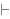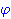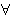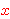New Foundations Explorer < Previous   Next > Nearby theorems Mirrors  >  Home  >  NFE Home  >  Th. List  >  ax17o Unicode version

Theorem ax17o 2157
 Description: Axiom to quantify a variable over a formula in which it does not occur. Axiom C5 in [Megill] p. 444 (p. 11 of the preprint). Also appears as Axiom B6 (p. 75) of system S2 of [Tarski] p. 77 and Axiom C5-1 of [Monk2] p. 113. (This theorem simply repeats ax-17 1616 so that we can include the following note, which applies only to the obsolete axiomatization.) This axiom is logically redundant in the (logically complete) predicate calculus axiom system consisting of ax-gen 1546, ax-5o 2136, ax-4 2135, ax-7 1734, ax-6o 2137, ax-8 1675, ax-12o 2142, ax-9o 2138, ax-10o 2139, ax-13 1712, ax-14 1714, ax-15 2143, ax-11o 2141, and ax-16 2144: in that system, we can derive any instance of ax-17 1616 not containing wff variables by induction on formula length, using ax17eq 2183 and ax17el 2189 for the basis together hbn 1776, hbal 1736, and hbim 1817. However, if we omit this axiom, our development would be quite inconvenient since we could work only with specific instances of wffs containing no wff variables - this axiom introduces the concept of a setvar variable not occurring in a wff (as opposed to just two setvar variables being distinct). (Contributed by NM, 19-Aug-2017.) (New usage is discouraged.) (Proof modification discouraged.)
Assertion
Ref Expression
ax17oDistinct variable group:,Proof of Theorem ax17o
StepHypRef Expression
1 ax-17 1616 1Colors of variables: wff setvar class Syntax hints:wi 4wal 1540 This theorem was proved from axioms:  ax-17 1616 This theorem is referenced by: (None)
 Copyright terms: Public domain W3C validator